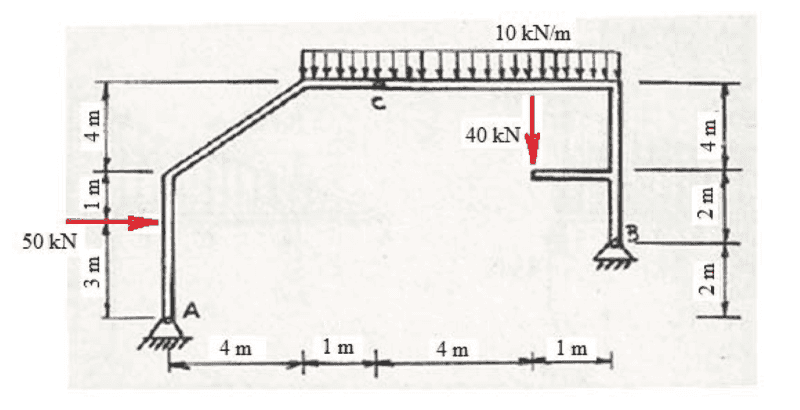# Static structure - find a fourth relation

Homework Statement:
As shown in the figure, we are given a static structure with articulated supports A and B. C is a point articulated too. We are asked to find the reaction forces and moments in A and B.
Relevant Equations:
##F_r = ma##, ##M_r = I\alpha##First, since A and B are articulated, the moments due to A and B are zero. Now, we may call reaction forces in A, ##V_A## and ##H_A## and in the same way, call the reactions in B as ##V_B## and ##H_B##. With that and Newton's third law, I managed to find three equations (equilibrium of translation in the vertical and in the horizontal plus the rotation equilibrium), but, still, I need one more equation or argument to completely find the four forces.

Lnewqban
Gold Member
You can remove one support at a time and calculate the forces that are needed to keep the structure from rotating about the other.
It is bassicaly an ABC triangular closed structure.

•Moara
I don't think I understand how am I supposed to remove one support, could you clarify, please? Meanwhile, I tried to split the structure looking only at the branch AC, can I say that in C there will be only horizontal forces, hence finding that ##V_A = 10 \ kN## ?

haruspex
Homework Helper
Gold Member
2020 Award
plus the rotation equilibrium
There are two components that, in principle, can rotate independently, so you should have two rotation equilibrium equations.

There are two components that, in principle, can rotate independently, so you should have two rotation equilibrium equations.
with that in mind, I did the equilibrium of rotation looking to C, from bar AC, getting another equation and finding ##H_A = \frac{90}{7}##, is it correct doing that ?

with that in mind, I did the equilibrium of rotation looking to C, from bar AC, getting another equation and finding ##H_A = \frac{90}{7}##, is it correct doing that ?
It appears that I have to look AC and BC separately right

with that in mind, I did the equilibrium of rotation looking to C, from bar AC, getting another equation and finding ##H_A = \frac{90}{7}##, is it correct doing that ?
It appears that I have to look AC and BC separately right

haruspex
Homework Helper
Gold Member
2020 Award
with that in mind, I did the equilibrium of rotation looking to C, from bar AC, getting another equation and finding ##H_A = \frac{90}{7}##, is it correct doing that ?
How do you get 90/7?

How do you get 90/7?
I probably did a mistake in my calculations

I probably did a mistake in my calculations
but I think that horizontal and vertical equilibrium plus rotation equilibrium of AC and BC around C is correct

haruspex